| Next|| Previous || Top |

# SOLID_SOLUTIONS

This keyword data block is used to define a solid-solution assemblage. Each solid solution may be nonideal with two components or ideal with any number of components. The amount of each component in each solid solution is defined in this keyword data block. Any calculation involving solid solutions assumes that all solid solutions dissolve entirely and reprecipitate in equilibrium with the solution.

### Example data block

```Line 0:  SOLID_SOLUTIONS 1 Two solid solutions
Line 1a:       CaSrBaSO4    # greater than 2 components, ideal
Line 2a:            -comp   Anhydrite   1.500
Line 2b:            -comp   Celestite   0.05
Line 2c:            -comp   Barite      0.05
Line 1b:       Ca(x)Mg(1-x)CO3    # Binary, nonideal
Line 3:            -comp1   Calcite          0.097
Line 4:            -comp2   Ca.5Mg.5CO3      0.003
Line 5:            -temp    25.0
Line 6:            -tempk   298.15
Line 7:            -Gugg_nondim   5.08       1.90
```

Optional definitions of excess free-energy parameters for nonideal solid solutions:

```Line 8:            -Gugg_kj          12.593  4.70
Line 9:            -activity_coefficients 24.05 1075. 0.0001 0.9999
Line 10:           -distribution_coefficients .0483 1248. .0001 .9999
Line 11:           -miscibility_gap  0.0428  0.9991
Line 12:           -spinodal_gap     0.2746  0.9483
Line 13:           -critical_point   0.6761  925.51
Line 14:           -alyotropic_point 0.5768  -8.363
Line 15:           -Thompson         17.303  7.883
Line 16:           -Margules         -0.62   7.6```

### Explanation

Line 0: SOLID_SOLUTIONS [ number ] [ description ]

SOLID_SOLUTIONS is the keyword for the data block. Optionally, SOLID_SOLUTION.

number --Positive number to designate the following solid-solution assemblage and its composition. A range of numbers may also be given in the form m-n , where m and n are positive integers, m is less than n , and the two numbers are separated by a hyphen without intervening spaces. Default is 1.

description --Optional comment that describes the surface assemblage.

Line 1: solid-solution name

solid-solution name --User-defined name of a solid solution.

Line 2: -comp phase name, moles

-comp--Identifier indicates a component of an ideal solid solution is defined. Component is part of the solid solution defined by the last Line 1 encountered. Optionally, comp, component, or -c[ omponent].

phase name --Name of the pure phase that is a component in the solid solution. A phase with this name must have been defined in a PHASES data block.

moles --Moles of the component in the solid solution.

Line 3: -comp1 phase name, moles

-comp1--Identifier indicates the first component of a nonideal, binary solid solution is defined. The component is part of the solid solution defined by the last Line 1 encountered. Optionally, comp1.

phase name --Name of the pure phase that is component 1 of the nonideal solid solution. A phase with this name must have been defined in a PHASES data block.

moles --Moles of the component in the solid solution.

Line 4: -comp2 phase name, moles

-comp2--Identifier indicates the second component of a nonideal, binary solid solution is defined. The component is part of the solid solution defined by the last Line 1 encountered. Optionally, comp2.

phase name --Name of the pure phase that is component 2 of the nonideal solid solution. A phase with this name must have been defined in a PHASES data block.

moles --Moles of the component in the solid solution.

Line 5: -temp temperature in Celsius

-temp--Temperature at which excess free-energy parameters are defined, in Celsius. Temperature, either temp ( tempc) or tempk, is used if excess free-energy parameters are input with any of the following options: -gugg_nondim, -activity_coefficients, -distribution_coefficients, -miscibility_gap, -spinodal_gap, -alyotropic_point, or -margules. Optionally, temp, tempc, or -t[ empc]. Default is 25 o C.

Line 6: -tempk temperature in Kelvin

-tempk--Temperature at which excess free-energy parameters are defined, in Kelvin. Temperature, either temp ( tempc) or tempk, is used if excess free-energy parameters are input with any of the following options: -gugg_nondim, -activity_coefficients, -distribution_coefficients, -miscibility_gap, -spinodal_gap, -alyotropic_point, or -margules. Optionally, tempk. Default is 298.15 K.

Line 7: -Gugg_nondim a 0 , a 1

-Gugg_nondim--Nondimensional Guggenheim parameters are used to calculate dimensional Guggenheim parameters. Optionally, gugg_nondimensional, parms, -g[ ugg_nondimensional], or -p[ arms].

a 0 --Guggenheim a 0 parameter, dimensionless. Default is 0.0.

a 1 --Guggenheim a 1 parameter, dimensionless. Default is 0.0.

Line 8: -Gugg_kJ g 0 , g 1

-Gugg_kJ--Guggenheim parameters with dimensions of kJ/mol define the excess free energy of the nonideal, binary solid solution. Optionally, gugg_kJ, or -gugg_k[ J].

g 0 --Guggenheim a 0 parameter, kJ/mol. Default is 0.0.

g 1 --Guggenheim a 1 parameter, kJ/mol. Default is 0.0.

Line 9: -activity_coefficients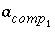,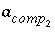, x 1 , x 2

-activity_coefficients--Activity coefficients for components 1 and 2 are used to calculate dimensional Guggenheim parameters. Optionally, activity_coefficients, or -a[ ctivity_coefficients].--Activity coefficient for component 1 in the solid solution. No default.--Activity coefficient for component 2 in the solid solution. No default.

x 1 --Mole fraction of component 2 for whichapplies. No default.

x 2 --Mole fraction of component 2 for whichapplies. No default.

Line 10: -distribution_coefficients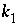,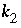, x 1 , x 2

-distribution_coefficients--Two distribution coefficients are used to calculate dimensional Guggenheim parameters. Optionally, distribution_coefficients, or -d[ istribution_coefficients].--Distribution coefficient of component 2 at mole fraction x 1 of component 2, expressed as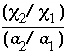, where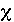is the mole fraction in the solid and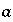is the aqueous activity. No default.--Distribution coefficient of component 2 at mole fraction x 2 of component 2, expressed as above. No default.

x 1 --Mole fraction of component 2 for whichapplies. No default.

x 2 --Mole fraction of component 2 for whichapplies. No default.

Line 11: -miscibility_gap x 1 , x 2

-miscibility_gap--The mole fractions of component 2 that delimit the miscibility gap are used to calculate dimensional Guggenheim parameters. Optionally, miscibility_gap, or -m[ iscibility_gap].

x 1 --Mole fraction of component 2 at one end of the miscibility gap. No default.

x 2 --Mole fraction of component 2 at the other end of the miscibility gap. No default.

Line 12: -spinodal_gap x 1 , x 2

-spinodal_gap--The mole fractions of component 2 that delimit the spinodal gap are used to calculate dimensional Guggenheim parameters. Optionally, spinodal_gap, or -s[ pinodal_gap].

x 1 --Mole fraction of component 2 at one end of the spinodal gap. No default.

x 2 --Mole fraction of component 2 at the other end of the spinodal gap. No default.

Line 13: -critical_point x cp , t cp

-critical_point--The mole fraction of component 2 at the critical point and the critical temperature (Kelvin) are used to calculate dimensional Guggenheim parameters. Optionally, critical_point, or -cr[ itical_point].

x cp --Mole fraction of component 2 at the critical point. No default.

t cp --Critical temperature, in Kelvin. No default.

Line 14: -alyotropic_point x aly ,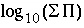-alyotropic_point--The mole fraction of component 2 at the alyotropic point and the total solubility product at that point are used to calculate dimensional Guggenheim parameters. Optionally, alyotropic_point, or -al[ yotropic_point].

x aly --Mole fraction of component 2 at the alyotropic point. No default.--Total solubility product at the alyotropic point, where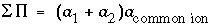. No default.

Line 15: -Thompson wg 2 , wg 1

-Thompson--Thompson and Waldbaum parameters wg 2 and wg 1 are used to calculate dimensional Guggenheim parameters. Optionally, thompson, or -th[ ompson].

wg 2 --Thompson and Waldbaum parameter wg 2 , kJ/mol. No default.

wg 1 --Thompson and Waldbaum parameter wg 1 , kJ/mol. No default.

Line 16: -Margules alpha 2 , alpha 3

-Margules--Margules parameters alpha 2 and alpha 3 are used to calculate dimensional Guggenheim parameters. Optionally, Margules, or -Ma[ rgules].

alpha 2 --Margules parameter alpha 2 , dimensionless. No default.

alpha 3 --Margules parameter alpha 3 , dimensionless. No default.

### Notes

Multiple solid solutions may be defined by multiple sets of lines 1, 2, 3, and 4. Line 2 may be repeated as necessary to define all the components of an ideal solid solution. Nonideal solid solution components must be defined with Lines 3, and 4. Calculations with solid solutions assume that the entire solid recrystallizes to be in equilibrium with the aqueous phase. This assumption is usually unrealistic because it is likely that only the outer layer of a solid would re-equilibrate with the solution, even given long periods of time. In most cases, the use of ideal solid solutions is also unrealistic because nonideal effects are nearly always present in solids.

Lines 7-16 provide alternative ways of defining the excess free energy of a nonideal, binary solid solution. Only one of these lines should be included in the definition of a single solid solution. The parameters in the example data block are taken from Glynn (1991) and Glynn (1990) for "nondefective" calcite (log K -8.48) and dolomite (expressed as Ca 0.5 Mg 0.5 CO 3 , log K -8.545; note that a phase for dolomite with the given name, composition, and log K would have to be defined in a PHASES data block because it differs from the standard stoichiometry for dolomite in the databases). In the example data block, lines 7 through 16, except Line 14 (alyotropic point), define the same dimensional Guggenheim parameters. Internally, the program converts any one of these forms of input into dimensional Guggenheim parameters. When a batch-reaction or transport calculation is performed, the temperature of the calculation (as defined by mixing of solutions, REACTION_TEMPERATURE data block, or heat transport in TRANSPORT simulations) is used to convert the dimensional Guggenheim parameters to nondimensional Guggenheim parameters, which are then used in the calculation.

The identifiers -gugg_nondim, -activity_coefficients, -distribution_coefficients, -miscibility_gap, -spinodal_gap, -alyotropic_point, or -margules define parameters for a particular temperature which are converted to dimensional Guggenheim parameters using the default temperature of 25 C or the temperature specified in line 5 or 6. If more than one line 5 and (or) 6 is defined, the last definition will take precedence. If -alyotropic_point or -distribution_coefficients identifiers are used to define excess free-energy parameters, the dimensional Guggenheim parameter are dependent on (1) the values included with these two identifiers and (2) the equilibrium constants for the pure-phase components. The latter are defined by a PHASES data block in the input file or database file.

The parameters for excess free energy are dependent on which component is labeled "1" and which component is labeled "2". It is recommended that the component with the smaller value of log K be selected as component 1 and the component with the larger value of log K be selected as component 2. The excess free-energy parameters must be consistent with this numbering. A positive value of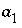(nondimensional Guggenheim parameter) or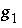(dimensional Guggenheim parameter) will result in skewing the excess free-energy function toward component 2 and, if a miscibility gap is present, it will not be symmetric about a mole fraction of 0.5, but instead will be shifted toward component 2. In the calcite-dolomite example, the positive value of(1.90) results in a miscibility gap extending almost to pure dolomite (mole fractions of miscibility gap are 0.0428 to 0.9991).

After a batch-reaction with a solid-solution assemblage has been simulated, it is possible to save the resulting solid-solution compositions with the SAVE keyword. If the new compositions are not saved, the solid-solution compositions will remain the same as they were before the batch reaction. After it has been defined or saved, the solid-solution assemblage may be used in subsequent simulations through the USE keyword. Solid-solution compositions are automatically saved following each shift in transport calculations.

### Example problems

The keyword SOLID_SOLUTIONS is used in example problem 10.

### Related keywords

PHASES, SAVE solid_solution, and USE solid_solution.

| Next|| Previous || Top |